# 第一次试验

|实验课程|机器学习|
| ---- | ---- | ---- |
|实验要求|点击查看|
|实验名称|感知器及其应用|
|学 号|3180701333|

1.理解感知器算法原理，能实现感知器算法；

2.掌握机器学习算法的度量指标；

3.掌握最小二乘法进行参数估计基本原理；

4.针对特定应用场景及数据，能构建感知器模型并进行预测。

1.安装Pycharm，注册学生版。

2.安装常见的机器学习库，如Scipy、Numpy、Pandas、Matplotlib，sklearn等。

3.编程实现感知器算法。

4.熟悉iris数据集，并能使用感知器算法对该数据集构建模型并应用。

1.按实验内容撰写实验过程；

2.报告中涉及到的代码，每一行需要有详细的注释；

3.按自己的理解重新组织，禁止粘贴复制实验内容！

import pandas as pd
import numpy as np
import matplotlib.pyplot as plt
#Matplotlib是Python的一个绘图库，是Python中最常用的可视化工具之一，可以非常方便地创建2D图表和一些基本的3D图表pyplot模块的plot函数可以接收输入参数和输出参数，还有线条粗细等参数
%matplotlib inline

# load data
df = pd.DataFrame(iris.data, columns=iris.feature_names) #是一个表格
df['label'] = iris.target # 表头字段就是key
df.plot(figsize = (12, 8)) # 利用dataframe做简单的可视化分析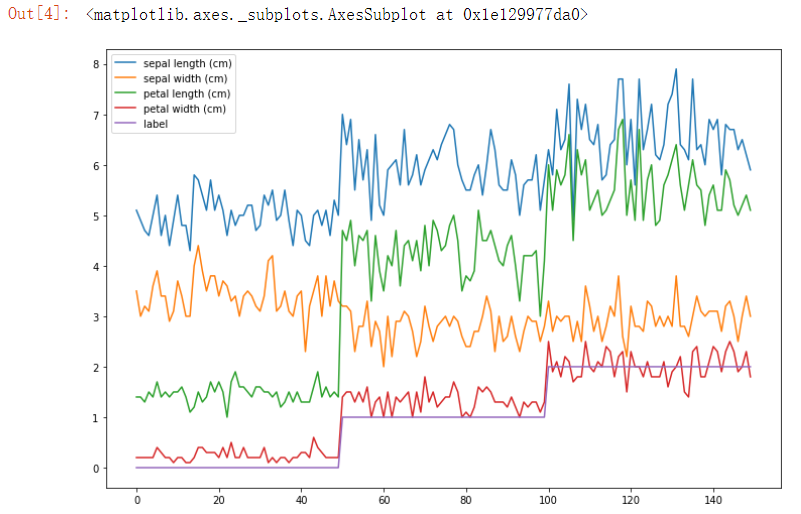df.columns = ['sepal length', 'sepal width', 'petal length', 'petal width', 'label'] # 选择其中的4个特征进行训练
df.label.value_counts()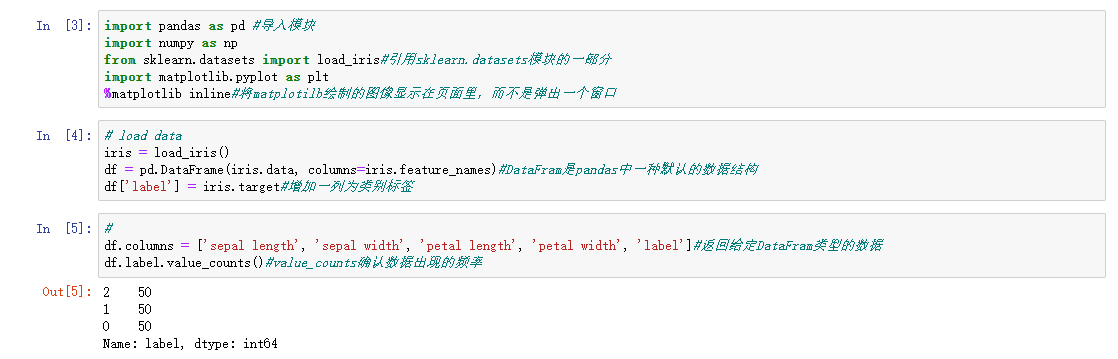plt.scatter(df[:50]['sepal length'], df[:50]['sepal width'], label='0')
plt.scatter(df[50:100]['sepal length'], df[50:100]['sepal width'], label='1')
plt.xlabel('sepal length')
plt.ylabel('sepal width')
plt.legend()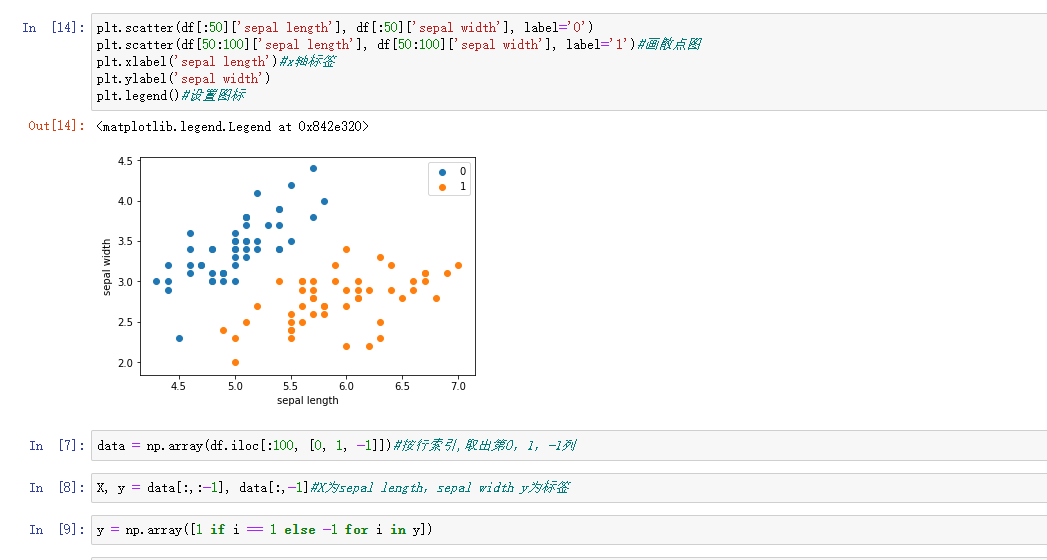data = np.array(df.iloc[:100, [0, 1, -1]]) # 取前100条数据，为了方便展示，取2个特征
X, y = data[:,:-1], data[:,-1]  # 数据类型转换，为了后面的数学计算
y = np.array([1 if i == 1 else -1 for i in y])

# 数据线性可分，二分类数据
# 此处为一元一次线性方程
class Model:
def __init__(self):
self.w = np.ones(len(data)-1, dtype=np.float32)
self.b = 0 #初始w/b的值
self.l_rate = 0.1
# self.data = data
def sign(self, x, w, b):
y = np.dot(x, w) + b #求w，b的值
#Numpy中dot()函数主要功能有两个：向量点积和矩阵乘法。
#格式：x.dot(y) 等价于 np.dot(x,y) ———x是m*n 矩阵 ，y是n*m矩阵，则x.dot(y) 得到m*m矩阵
return y

# 随机梯度下降法
#随机梯度下降法（SGD），随机抽取一个误分类点使其梯度下降。根据损失函数的梯度，对w，b进行更新
def fit(self, X_train, y_train): #将参数拟合 X_train数据集矩阵 y_train特征向量
is_wrong = False
#误分类点的意思就是开始的时候，超平面并没有正确划分，做了错误分类的数据。
while not is_wrong:
wrong_count = 0 #误分为0，就不用循环，得到w，b
for d in range(len(X_train)):
X = X_train[d]
y = y_train[d]
if y * self.sign(X, self.w, self.b) <= 0:
# 如果某个样本出现分类错误，即位于分离超平面的错误侧，则调整参数，使分离超平面开始移动，直至误分类点被正确分类。
self.w = self.w + self.l_rate*np.dot(y, X) #调整w和b
self.b = self.b + self.l_rate*y
wrong_count += 1
if wrong_count == 0:
is_wrong = True
return 'Perceptron Model!'
#线性可分可用随机梯度下降法
def score(self):
pass

# 拟合
perceptron = Model()
perceptron.fit(X, y)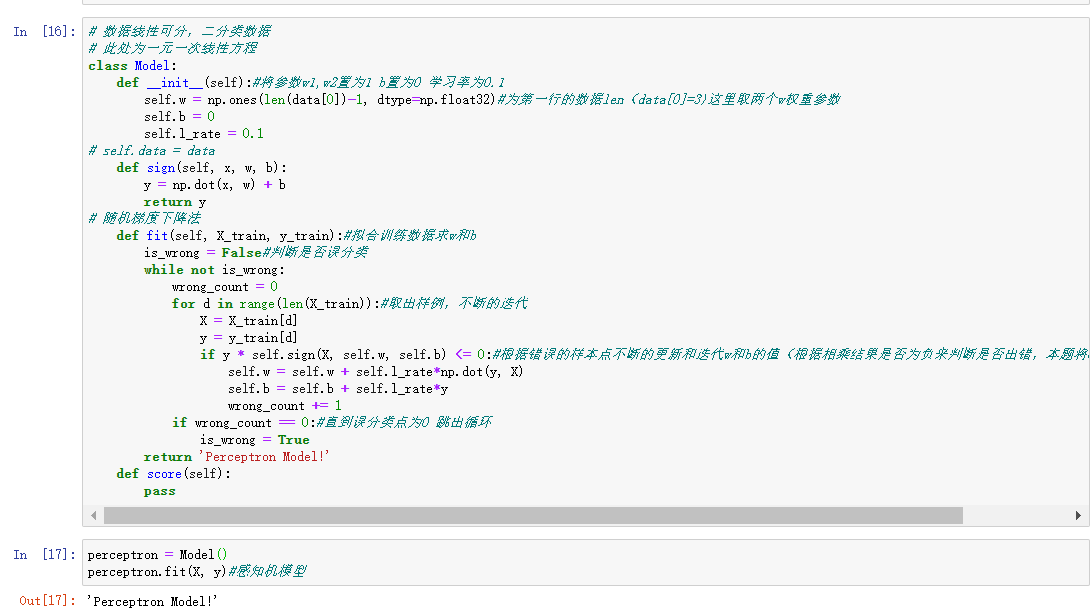x_points = np.linspace(4, 7,10)
y_ = -(perceptron.w*x_points + perceptron.b)/perceptron.w
plt.plot(x_points, y_)
plt.plot(data[:50, 0], data[:50, 1], 'bo', color='blue', label='0')
plt.plot(data[50:100, 0], data[50:100, 1], 'bo', color='orange', label='1')
plt.xlabel('sepal length')
plt.ylabel('sepal width')
plt.legend()

#定义感知机
from sklearn.linear_model import Perceptron

#使用训练数据进行训练
clf = Perceptron(fit_intercept=False, max_iter=1000, shuffle=False)

#得到训练结果，权重矩阵
clf.fit(X,y)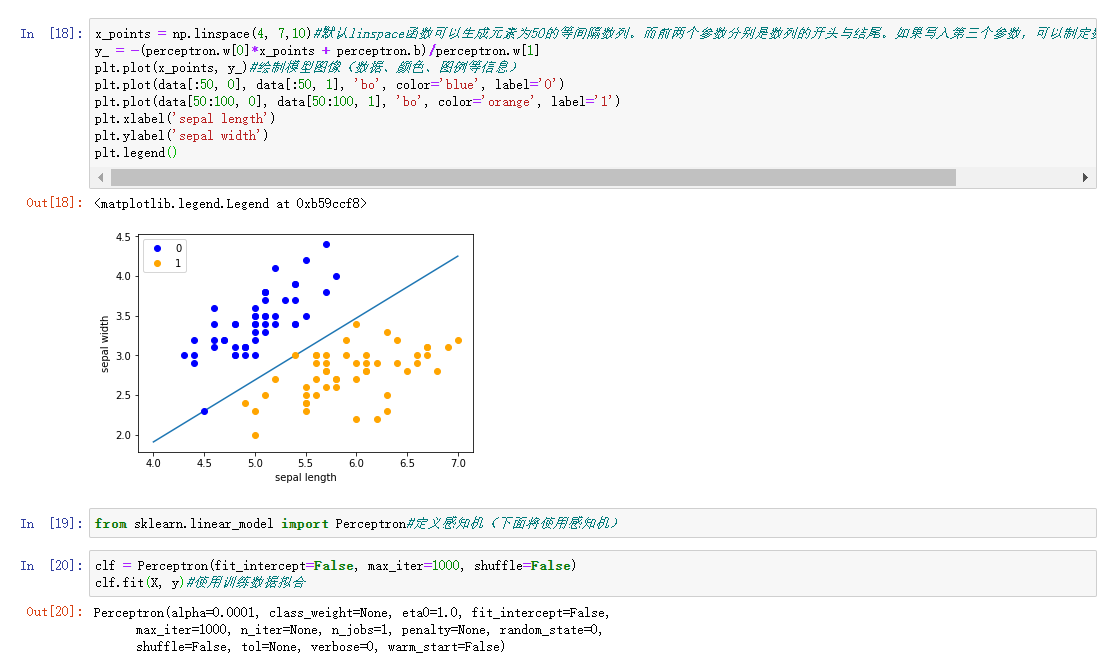# Weights assigned to the features.输出特征权重矩阵
print(clf.coef_)

# 超平面的截距 Constants in decision function.
print(clf.intercept_)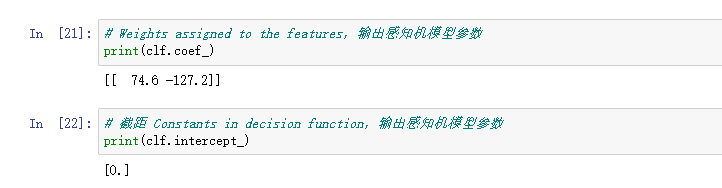posted @ 2021-05-10 10:38  秃头=变强786  阅读(62)  评论(0编辑  收藏  举报